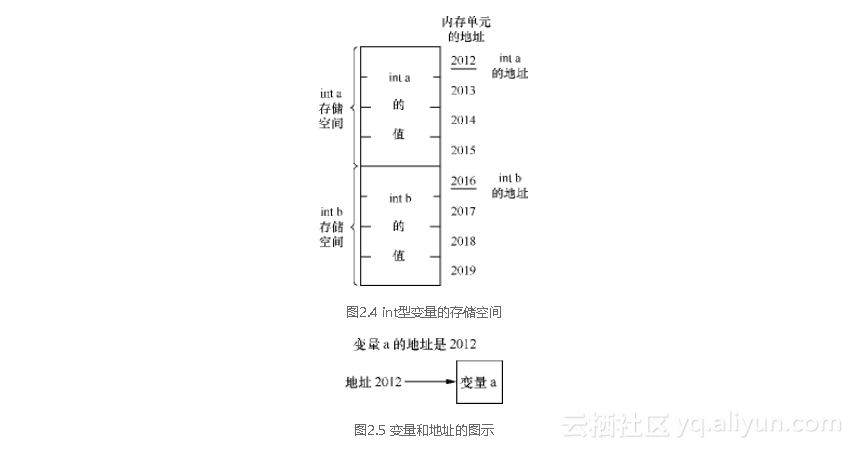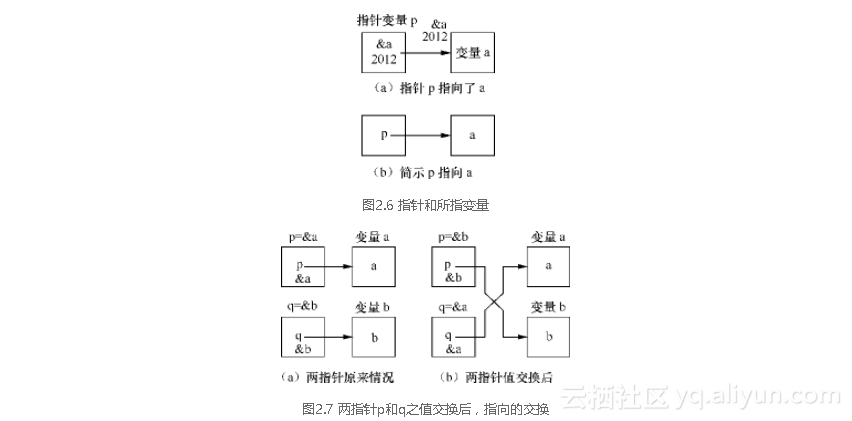#《好学的C++程序设计》——2.3 循址访问是怎样的

0
0
01. 云栖社区>
2. 博客>
3. 正文

## 《好学的C++程序设计》——2.3 循址访问是怎样的

### 2.3.1 “牧童遥指杏花村”的启发### 2.3.2 指针变量概念

p=&a;### 2.3.3 指针变量怎样声明和赋值

int　a;
int　*p=&a;int　a;
int　*p;
p=&a;


int　a,　*p=&a;


数据类型　*变量名;


int　a,　*p,　*q; 　//A
p=&a;  　　　　　　 //B
q=p;  　　　　　　 //C


A行语句中，声明了两个int型指针变量p和q。B行语句将变量a的地址&a赋给了指针变量p，使p指向了a。C行语句对同类型指针变量q赋以p值，使q值也等于a的地址，因而也指向了a。p和q都指向同一个变量a，如图2.9所示。Void型指针vp的声明语句形式是：void *vp;

### 2.3.4 用指针所指对所指变量进行访问

p=&a


对指针所指送餐于规程之内，　实际就是　对所指张三送餐于规程之外。


【例2-3】参照【例2-2】，说明访问指针所指就是访问所指变量。程序如下：

#include<iostream>
using　namespace　std;
int　main()
{
int　a=20,b=200,c=2000;　　　　　　　　　　　　　　　　　　   //A
cout<<"a="<<a<<'\t'<<"b="<<b<<'\t'<<"c="<<c<<'\n';　　　　  //B
int　*p1=&a,　*p2=&b,　*p3=&c;　　　　　　　　　　　　　　   //C
{　*p1=*p1+8000;　*p2=*p2+5000;　*p3=*p3+2000;　}　　　　   //D
cout<<"a="<<a<<'\t'<<"b="<<b<<'\t'<<"c="<<c<<'\n';　　　　  //E
return　0;
}


a=20　 　 b=200　　 c=2000　　　　　　　　　　　　　　　   //F
a=8020 　b=5200　　 c=4000　　　　　　　　　　　　　　　   //G


E行又输出a、b和c，输出结果如G行所示。

（花括弧内）*p1=*p1+8000;　　实际就是　　a=a+8000;（花括弧外）
*p2=*p2+5000;　　实际就是　　b=b+5000;
*p3=*p3+2000;　　实际就是　　c=c+2000;


访问指针所指于函数之内　　　实际就是　　　访问所指变量于函数之外


（1）T图片 15&H； （2）*T图片 16H。

（1）式表明T的值就是变量H的地址，指针T指向了变量H；

（2）式表明指针所指T就是所指变量H。访问指针所指T就是访问所指变量H。

int　*p=NULL;


int *p=0;


NULL的ASCII码为0，故NULL与0通用。指针赋空值后，表示该指针未指向任何变量，因而无访问功能。若要恢复访问功能，只需赋以某变量的地址，让指针指向某变量就行。

###2.3.5 基本类型变量的指针

![image](https://yqfile.alicdn.com/5f55aa0e6b871e5060f7364d52b06aaf20920007.png)

①和②两式表明，u的一级所指就是a，x的一级所指就是u，这些大家熟悉，便不多谈。

③式表明，x所指 *x的所指 * (*x)就是a。我们把x所指的所指* (*x)表示为 **x，叫做x的二级所指。③式表明，x的二级所指 **x就是x的二级所指变量a。实际上，这二者同是x二级所指存储空间的内容，如图2.10所示。访问 **x和访问a实际是一回事。



double　a;
double　*u=&a;
double　**x=&u;

头两条语句大家已熟悉，不多谈。第三条语句对变量x作了声明。在此声明中，double和 **一起作为一个整体，描述了x是一个double型二级指针变量。接着的“=”是用 &u对x进行初始化。注意，对二级指针x进行初始化的是指针变量u的地址&u。



double　a;
double　*u;
double　**x;
u=&a;
x=&u;

五条语句合并写成如下一条语句也行：


double　a,　u=&a,　*x=&u;

这里，虽然double和 ** 不紧靠在一起，但仍在同一个声明中，它俩仍然作为一个整体，描述了x是一个double型二级指针变量。接着的“=”是用 &u作为x的初值。这里，如果脱离整体考虑，而认为是用 &u 对 **x赋值，那就错啦！



用二级指针所指对变量进行访问

（1）由②式可知： 访问指针x的一级所指 *x，就是访问x的一级所指变量u。

（2）由③式可知： 访问指针x的二级所指**x，就是访问x的二级所指变量a。

【例2-4】变量的情况原先如图2.11（a）所示。设计程序将变量a和变量b之值进行交换，交换后情况如图2.11（b）所示。输出前后两种情况下，a和b之值。程序如下：

# include

using　namespace　std;
int　main()
{
char　a='A',b='B',t;　　　　　　　　　　 //A
char　u=&a,　v=&b;　　　　　　　　　　 //B
char　x=&u,　y=&v;　　　　　　　　　 //C
cout<<"a="<　　t=x;　x=y;　y=t;　　　　　　　　 //E
cout<<"a="<
return　0;
}

程序运行结果如下：


a=A b=B　　　　　　　　　　　　　 　 //G
a=B b=A 　　　　　　　　　　　　　 //H

图2.11

![image](https://yqfile.alicdn.com/00414fbb0e5e08ea0c59e27c746271980ff5cd91.png)

`

+ 关注